# Index transform

An integral transform whose kernel (cf. also Kernel of an integral operator) depends upon some of the indices (or parameters, subscripts) of the special functions that participate in its definition. Such transforms are of non-convolution type and, as a rule, inversion formulas for them contain different special functions while the integration takes place over subscripts of these.

Some of the main index transforms include the Kontorovich–Lebedev transform over the index of the Macdonald function, the Mehler–Fock transform over the index of the associated Legendre function of the first kind (cf. also Legendre functions), the Olevskii transform over the index of the Gauss hypergeometric function, and the Lebedev transform over the index of the square of the Macdonald function. The Lebedev–Skal'skaya transform, over the index of the real (imaginary) parts of the Macdonald functions, is also worth mentioning here.

The theory of such transformations is still under construction. As can be shown, all these transforms can be represented by composition of the Kontorovich–Lebedev transform and some transform of Mellin type. Therefore, the Kontorovich–Lebedev transform plays a key role in such constructions.

By choosing different types of Mellin convolution transforms one can find new examples of index transforms. In this manner, J. Wimp discovered a pair of index transforms over the parameter of the Whittaker function: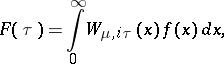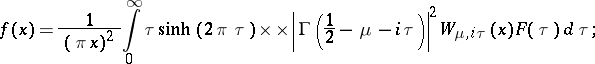J. Wimp [a3] introduced an index transform over parameters of the Meijer \$G\$-functions and he gave an inversion formula for which S.B. Yakubovich later obtained a more compact form: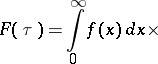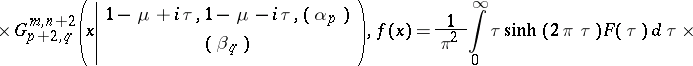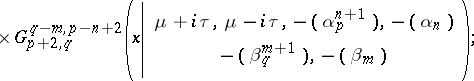E.C. Titchmarsh and Yakubovich discovered an index transform with a combination of Bessel and Lommel functions: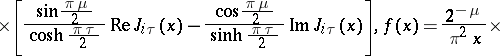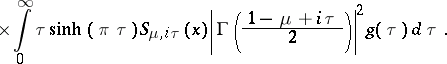For other index transforms, properties and applications, see [a2].

How to Cite This Entry:
Index transform. Encyclopedia of Mathematics. URL: http://encyclopediaofmath.org/index.php?title=Index_transform&oldid=28759
This article was adapted from an original article by S.B. Yakubovich (originator), which appeared in Encyclopedia of Mathematics - ISBN 1402006098. See original article